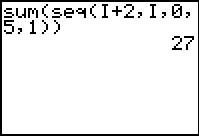# Knowledge Base

## Solution 11709: Calculating the Summation of a Sequence Using a TI-83 Family Graphing Calculator.

### How do I calculate the summation of a sequence using a TI-83 family graphing calculator?

The sum( and seq( functions can be combined in order to calculate the summation of a range of consecutive terms in a sequence. The necessary syntax is given below:

sum(seq(expression,variable,begin,end[,increment]))

The expression is evaluated for each value of the variable from begin to end, and returns the sum of the results. The following example demonstrates how to sum up the first n terms in an algebraic sequence.

For Example:

Evaluate Σ (I+2) from I=0 to I=5:

Solution:

1) On the home screen, press [2ND] [LIST] [Right Arrow] [Right Arrow]  to select 5:sum( List MATH Menu.
2) Press [2ND] [LIST] [Right Arrow]  to select 5:seq( from the List OPS Menu.
3) Input the expression [ALPHA] [I] [+]  then press [,].
4) Press [ALPHA] [I] to input the variable, then press [,].
5) Press  [,]  to input the low and high values, then press [,].
6) Press  to input the increment, then press [)] [)]. The display should now read "sum(seq(I+2,I,0,5,1))".
7) Press [ENTER]. The result of the summation is 27.